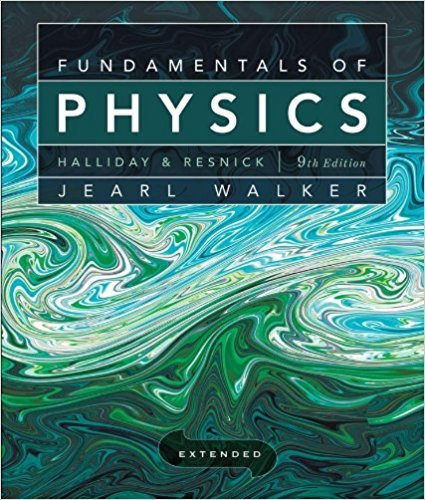# Solved: Figure lS-41 represents a closed cycle for a gas## Problem 49 Chapter 18

Fundamentals of Physics Extended | 9th Edition

• 2901 Step-by-step solutions solved by professors and subject experts
• Get 24/7 help from StudySoup virtual teaching assistantsFundamentals of Physics Extended | 9th Edition

4 5 0 255 Reviews
15
1
Problem 49

Figure lS-41 represents a closed cycle for a gas (the figure is not drawn to scale). The change in the in- p ternal energy of the gas as it moves from a to c along the path abc is -200 1. As it moves from c to d, lS0 J must B Volume Fig. 18-40 Problem4S. be transferred to it as heat. An additional transfer of SO J to it as heat is needed as it moves from d to a. How much work is done on the gas as it moves from c to d?

Step-by-Step Solution:
Step 1 of 3

Step 2 of 3

Step 3 of 3

##### ISBN: 9780470469088

Since the solution to 49 from 18 chapter was answered, more than 217 students have viewed the full step-by-step answer. Fundamentals of Physics Extended was written by and is associated to the ISBN: 9780470469088. The answer to “Figure lS-41 represents a closed cycle for a gas (the figure is not drawn to scale). The change in the in- p ternal energy of the gas as it moves from a to c along the path abc is -200 1. As it moves from c to d, lS0 J must B Volume Fig. 18-40 Problem4S. be transferred to it as heat. An additional transfer of SO J to it as heat is needed as it moves from d to a. How much work is done on the gas as it moves from c to d?” is broken down into a number of easy to follow steps, and 96 words. The full step-by-step solution to problem: 49 from chapter: 18 was answered by , our top Physics solution expert on 12/27/17, 08:15PM. This textbook survival guide was created for the textbook: Fundamentals of Physics Extended, edition: 9. This full solution covers the following key subjects: . This expansive textbook survival guide covers 37 chapters, and 3413 solutions.

Unlock Textbook Solution

Solved: Figure lS-41 represents a closed cycle for a gas

×
Get Full Access to Fundamentals Of Physics Extended - 9 Edition - Chapter 18 - Problem 49

Get Full Access to Fundamentals Of Physics Extended - 9 Edition - Chapter 18 - Problem 49

I don't want to reset my password

Need help? Contact support

Need an Account? Is not associated with an account
We're here to help